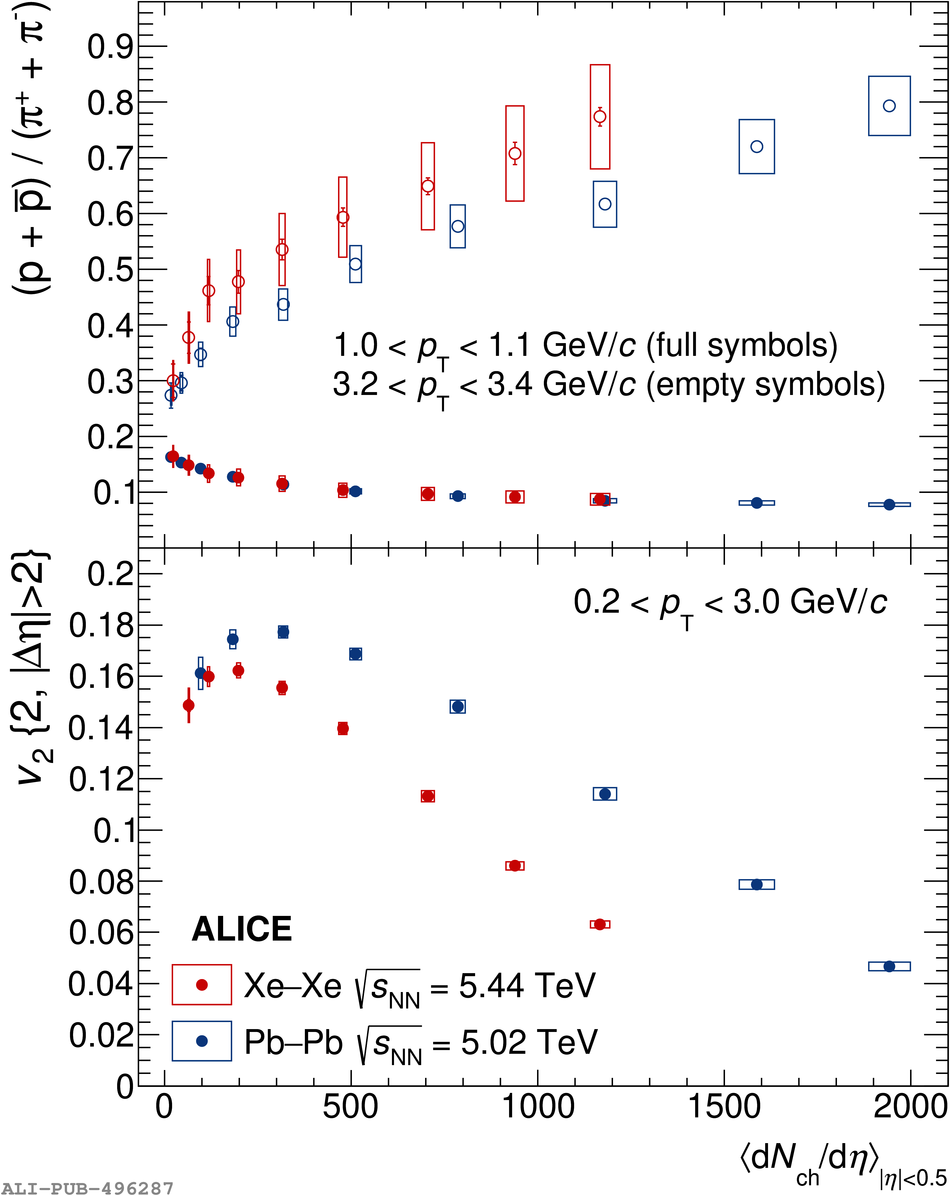Figure 5

 Proton-to-pion ratio as a function of charged particle multiplicity density in two \pt intervals for \xexe and \pbpb collisions at \snn~=~5.44 and 5.02 TeV In the bottom panel, the flow coefficient \vtwotwo is plotted for the same collision systems~ as a function of charged particle multiplicity density The statistical and systematic uncertainties are shown as error bars and boxes around the data points